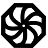# Programming-Idioms# Idiom #48 Multi-line string literal

Assign to variable s a string literal consisting in several lines of text

```auto s = ` line1
line2
line3`;

auto r = r" line1
line2
line3`;```
```auto s = "One,
Two,
Three
";```
```s : String := "Will this compile? " &
"Oh yes it will";```
```char *s = "Huey\n"
"Dewey\n"
"Louie";```
```(def s "Murs, ville,
Et port,
Asile
De mort,
Mer grise
Où brise
La brise,
Tout dort.")```
```IDENTIFICATION DIVISION.
PROGRAM-ID. multi-line string.
DATA DIVISION.
WORKING-STORAGE SECTION.
01 s    PIC X(20).
01 str  PIC X(5) VALUE 'COBOL'.
01 str1 PIC X(4) VALUE 'RULE'.
01 str2 PIC X(3) VALUE 'THE'.
01 str3 PIC X(5) VALUE 'WORLD'.
PROCEDURE  DIVISION.
STRING str ' ' str1 ' ' str2 ' ' str3
DELIMITED BY SIZE INTO s
STOP RUN.```
`#include <string>`
```std::string multiline = R"( Earth is a planet.
So is the Jupiter)";```
```string s = @"Huey
Dewey
Louie";```
```var s = '''A
multi-line
string''';```
```s = """
multiline
heredoc
"""```
```s = "Spanning
string
works"```
```S = "Strings
may span
across multiple lines"
"and they can"
"have as many portions"
"as you want"
"all of them quoted".```
```  s = "Hello &
&World"
```
```s := `Huey
Dewey
Louie````
```s = unlines [
"several"
,"lines"
,"of"
,"text"]```
```let s = "This is a very long string which needs \
to wrap across multiple lines because \
```let s = `This is a very long string which needs
to wrap across multiple lines because
```let s = "This is a very long string which needs " +
"to wrap across multiple lines because " +
```let s = `This is a very long string which needs
to wrap across multiple lines because
```String s = "This is a very long string which needs " +
"to wrap across multiple lines because " +
```val myStr =
"""
This is my
multi-line string.
"""```
```(setf s "a
b
c
d")```
```s = [[
Huey
Dewey
Louie
]]
```
```str = 'Huey '..
'Dewey '..
'Louie'```
```\$s = <<<EOD
Huey
Dewey
Louie
EOD;```
```\$s = "This string
is spanning
three lines";```
```var
_s: String;
begin
_s := 'one' + LineEnding + 'two' + LineEnding + 'three'
end.```
```\$s = "Perl normally allows
strings to contain newlines.";
\$s = q{
There are a good few ways to create multiline
text strings in perl.
};
\$s =<<EOSTR;
One of them is called the "here doc" (lifted from various UNIX shells).
A 'here doc' is the <<tag construct.  Perl continues to treat
all the text found as part of the string until there's a line containing
the EOSTR tag at the beginning
EOSTR```
```s = """Huey
Dewey
Louie"""```
```s = "Spanning
string
works"```
```let s = r#"Huey
Dewey
Louie"#;```
```let s = "line 1
line 2
line 3";```
```val s = """line 1
line 2
line 3"""```
```Dim myStr as String = "
This
Is
Multiline
Text!"```

deleplace